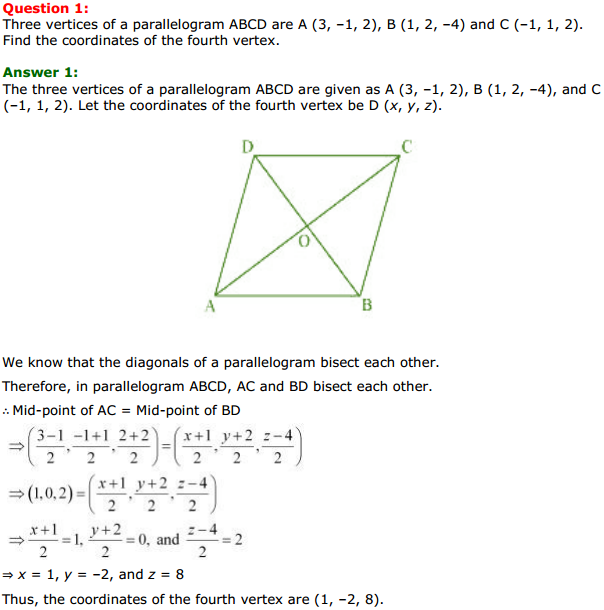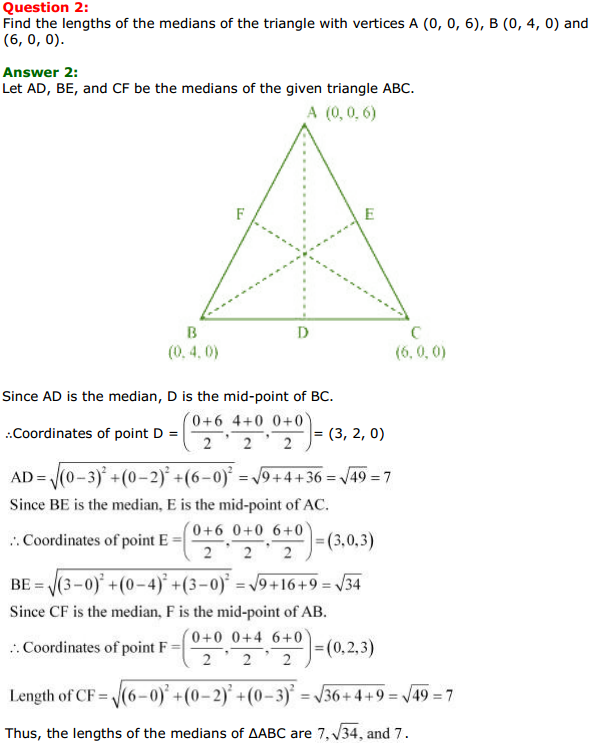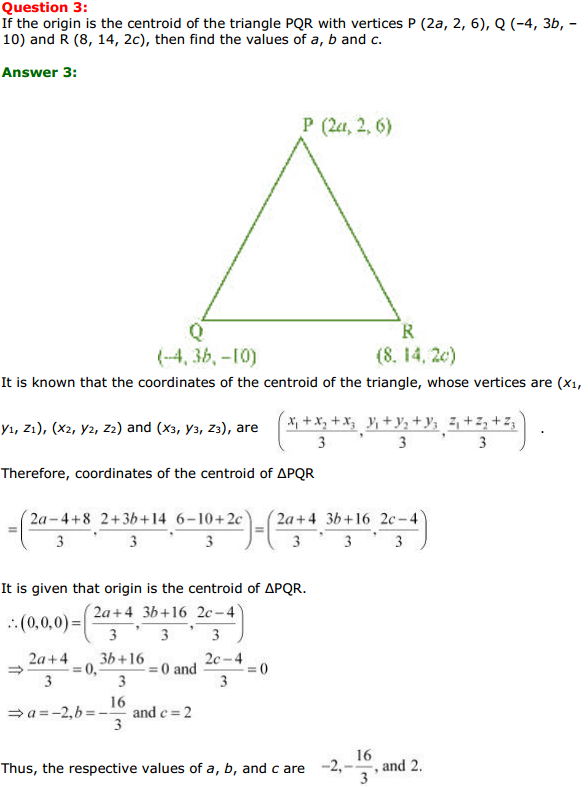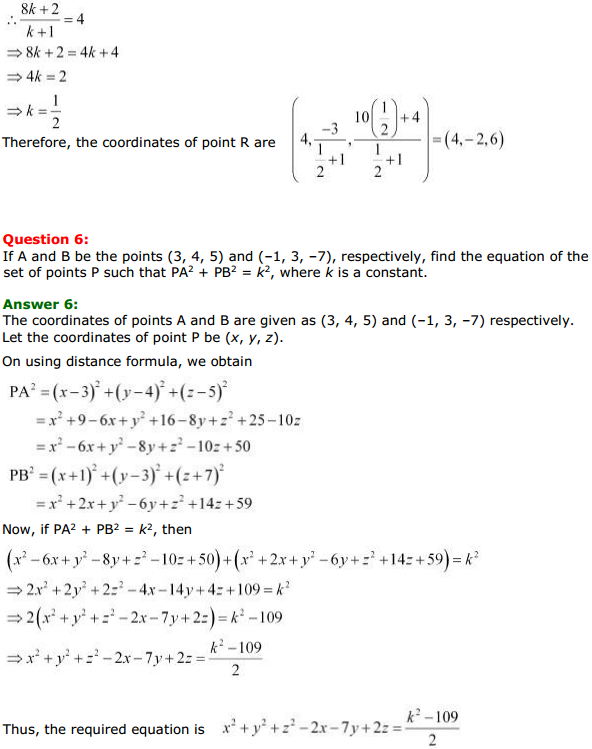Access Class 11 Maths NCERT Solutions Chapter 12 Introduction to three Dimensional Geometry Miscellaneous Exercise, contains solutions for all Introduction to three Dimensional Geometry Miscellaneous Exercise Class 11 questions.

## Introduction to three Dimensional Geometry Class 11 NCERT Solutions Maths Chapter 12 Introduction to three Dimensional Geometry Miscellaneous Exercise